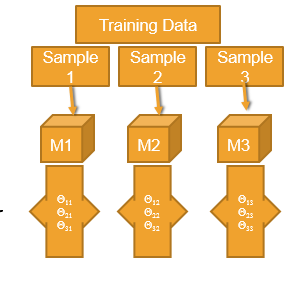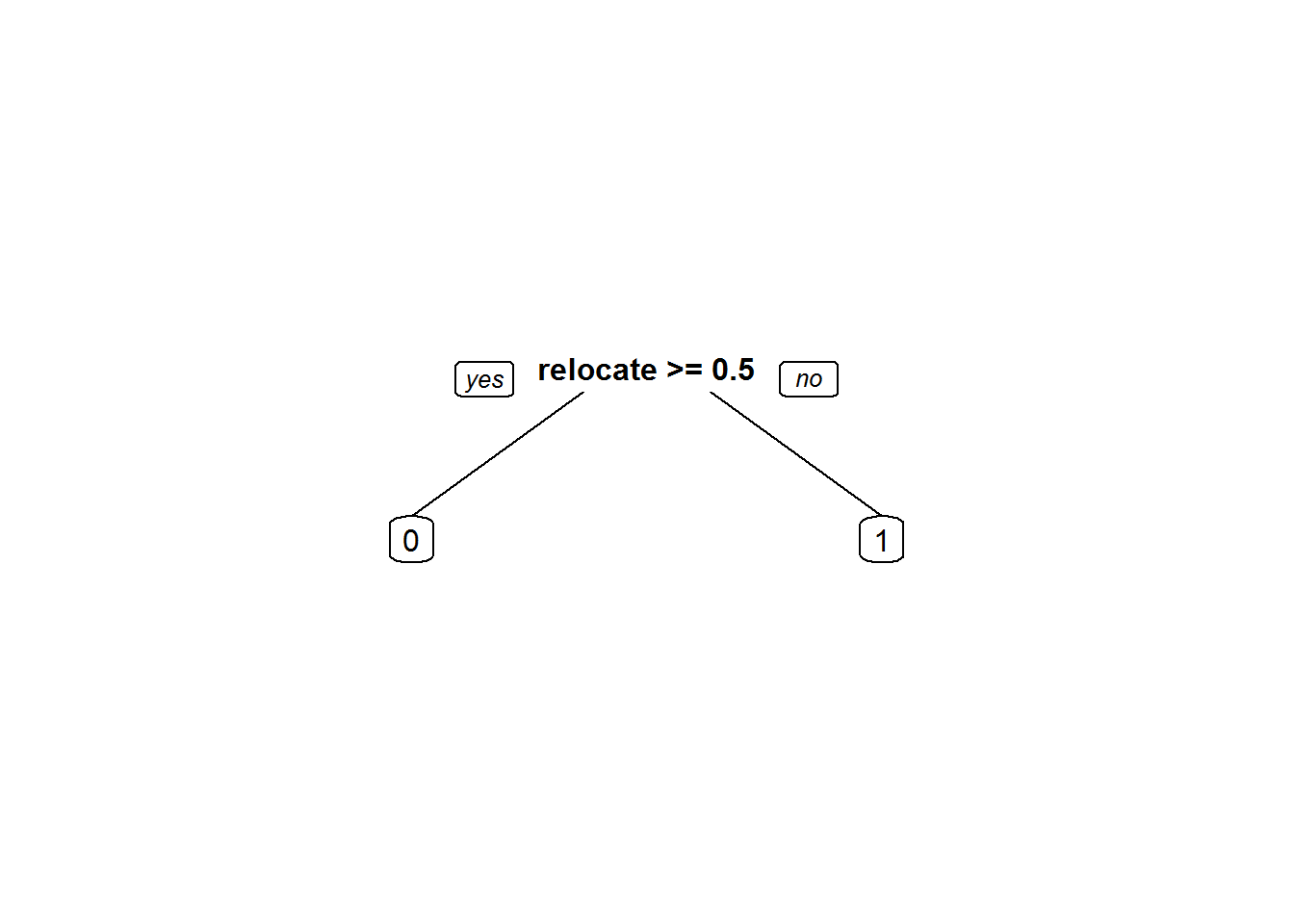• No products in the cart.

203.4.5 Type of Datasets, Type of Errors and Problem of Overfitting

The Problem of Over Fitting

In previous section, we studied about What is a Best Model?

• In search of the best model on the given data we add many predictors, polynomial terms, Interaction terms, variable transformations, derived variables, indicator/dummy variables etc.,
• Most of the times we succeed in reducing the error. What error is this?
• So by complicating the model we fit the best model for the training data.
• Sometimes the error on the training data can reduce to near zero
• But the same best model on training data fails miserably on test data.
• Imagine building multiple models with small changes in training data. The resultant set of models will have huge variance in their parameter estimates.• The model is made really complicated, that it is very sensitive to minimal changes
• By complicating the model the variance of the parameters estimates inflates
• Model tries to fit the irrelevant characteristics in the data
• Over fitting
• The model is super good on training data but not so good on test data
• We fit the model for the noise in the data
• Less training error, high testing error
• The model is over complicated with too many predictors
• Model need to be simplified
• A model with lot of variance

LAB: Model with huge Variance

• Data: Fiberbits/Fiberbits.csv
• Take initial 90% of the data. Consider it as training data. Keep the final 10% of the records for validation.
• Build the best model(5% error) model on training data.
• Use the validation data to verify the error rate. Is the error rate on the training data and validation data same?

Solution

fiber_bits_train<-Fiberbits[1:90000,]
fiber_bits_validation<-Fiberbits[90001:100000,]

Model on training data

Fiber_bits_tree3<-rpart(active_cust~., method="class", control=rpart.control(minsplit=5, cp=0.000001), data=fiber_bits_train)
Fbits_pred3<-predict(Fiber_bits_tree3, type="class")
conf_matrix3<-table(Fbits_pred3,fiber_bits_train\$active_cust)
accuracy3<-(conf_matrix3[1,1]+conf_matrix3[2,2])/(sum(conf_matrix3))
accuracy3
##  0.9524889

Validation Accuracy

fiber_bits_validation\$pred <- predict(Fiber_bits_tree3, fiber_bits_validation,type="class")

conf_matrix_val<-table(fiber_bits_validation\$pred,fiber_bits_validation\$active_cust)
accuracy_val<-(conf_matrix_val[1,1]+conf_matrix_val[2,2])/(sum(conf_matrix_val))
accuracy_val
##  0.7116

Error rate on validation data is more than the training data error.

The Problem of Under-fitting

• Simple models are better. Its true but is that always true? May not be always true.
• We might have given it up too early. Did we really capture all the information?
• Did we do enough research and future reengineering to fit the best model? Is it the best model that can be fit on this data?
• By being over cautious about variance in the parameters, we might miss out on some patterns in the data.
• Model need to be complicated enough to capture all the information present.
• If the training error itself is high, how can we be so sure about the model performance on unknown data?
• Most of the accuracy and error measuring statistics give us a clear idea on training error, this is one advantage of under fitting, we can identify it confidently.
• Under fitting
• A model that is too simple
• A mode with a scope for improvement
• A model with lot of bias

LAB: Model with huge Bias

• Lets simplify the model.
• Take the high variance model and prune it.
• Make it as simple as possible.
• Find the training error and validation error.

Solution

• Simple Model
Fiber_bits_tree4<-rpart(active_cust~., method="class", control=rpart.control(minsplit=30, cp=0.25), data=fiber_bits_train)
prp(Fiber_bits_tree4)Fbits_pred4<-predict(Fiber_bits_tree4, type="class")
conf_matrix4<-table(Fbits_pred4,fiber_bits_train\$active_cust)
conf_matrix4
##
## Fbits_pred4     0     1
##           0 11209   921
##           1 25004 52866
accuracy4<-(conf_matrix4[1,1]+conf_matrix4[2,2])/(sum(conf_matrix4))
accuracy4
##  0.7119444
• Validation accuracy
fiber_bits_validation\$pred1 <- predict(Fiber_bits_tree4, fiber_bits_validation,type="class")

conf_matrix_val1<-table(fiber_bits_validation\$pred1,fiber_bits_validation\$active_cust)
accuracy_val1<-(conf_matrix_val1[1,1]+conf_matrix_val1[2,2])/(sum(conf_matrix_val1))
accuracy_val1
##  0.4224